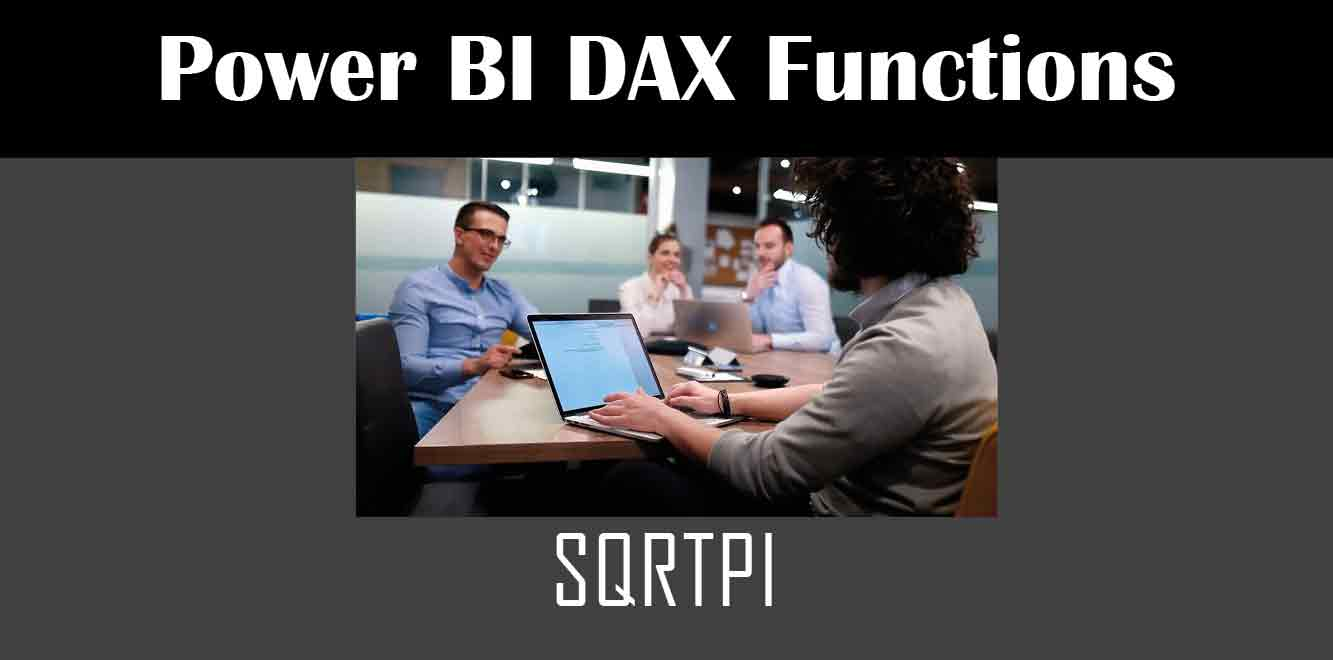# SQRTPI

## Y## What is the SQRTPI Function?

The SQRTPI function in Power BI DAX is a mathematical function that returns the square root of pi multiplied by a given number. This function allows for quick and easy calculations of complex equations without the need for advanced mathematical knowledge.

## How to Use the SQRTPI Function

Using the SQRTPI function in Power BI DAX is simple. To use the function, you must first enter it into the formula bar in the Power BI desktop. The syntax for the SQRTPI function is as follows:

SQRTPI(Number)

``` In this syntax, "Number" represents the value you wish to multiply by the square root of pi. For example, if you want to calculate the square root of pi multiplied by 10, you would enter the following formula: ```

SQRTPI(10)

``` Once you have entered the formula into the formula bar, press enter to calculate the result. Examples of Applications There are many applications for the SQRTPI function in Power BI DAX. One common use case is in financial modeling, where the function can be used to calculate the standard deviation of a portfolio of investments. Another use case is in scientific research, where the function can be used to calculate the surface area of a sphere. Let's look at an example of how the SQRTPI function can be used in Power BI DAX. Suppose you have a dataset containing sales data for a company. You want to calculate the square root of pi multiplied by the average sales for the year. To do this, you would enter the following formula into the formula bar: ```

SQRTPI(AVERAGE(Sales[SalesAmount]))

``` This formula would return the result of the square root of pi multiplied by the average sales for the year. The SQRTPI function in Power BI DAX is a powerful tool for simplifying complex calculations. Whether you are working in finance, science, or any other field that requires mathematical calculations, the SQRTPI function can make your work faster and easier. By following the simple syntax outlined above, you can quickly and easily use the SQRTPI function in Power BI DAX to perform a variety of calculations. Power BI DAX Training Courses by G Com Solutions (0800 998 9248)Power BI DAX Intensive Training Course £1,050.00 – £26,550.00 Select optionsContinue Loading DonePower BI DAX Introduction £395.00 – £9,750.00 Select optionsContinue Loading DonePower BI DAX Intermediate £395.00 – £9,750.00 Select optionsContinue Loading DonePower BI DAX Advanced £395.00 – £9,750.00 Select optionsContinue Loading Done Upcoming Courses Contact Us Subject Your Name (required) Company/Organisation Email (required) Telephone Training Course(s) Power BI Intensive TrainingPower BI introduction Power BI IntermediatePower BI AdvancedDAXPower Query MPower BI CertificationPower BI AdministrationPower PlatformPower AutomatePower AppsOTHER Your Message Upload Example Document(s) (Zip multiple files) ```
``` ```
``` ```
``` ```
``` ```
``` ```